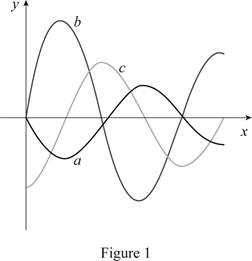# The graphs of f , f ′ and ∫ 0 x f ( t ) d t .### Single Variable Calculus: Concepts...

4th Edition
James Stewart
Publisher: Cengage Learning
ISBN: 9781337687805### Single Variable Calculus: Concepts...

4th Edition
James Stewart
Publisher: Cengage Learning
ISBN: 9781337687805

#### Solutions

Chapter 5, Problem 7RE
To determine

## To identify: The graphs of f,  f′ and ∫0xf(t)dt.

Expert Solution

• Curve (a) represents the integral function 0xf(t)dt.
• Curve (b) represents f.
• Curve (c) represents f.

### Explanation of Solution

Given information:

Show the graphs of f, f and 0xf(t)dt as in Figure 1.Calculation:

Refer to Figure 1.

Consider the explanation of graph as follows:

• f(t) is the double derivative of 0xf(t)dt.
• The integral function 0xf(t)dt is increases with positive value of double derivative function f(t).
• The value of double derivative function f(t) is increases with positive value of double derivative function f(t).

Curve (a) represents the integral function 0xf(t)dt, curve (b) represents the derivative of integral function f, and the curve (c) represents the double derivative of integral function f.

### Have a homework question?

Subscribe to bartleby learn! Ask subject matter experts 30 homework questions each month. Plus, you’ll have access to millions of step-by-step textbook answers!